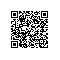# php 获取今日、昨日、上周、本月的起始时间戳和结束时间戳的方法

php 获取今日、昨日、上周、本月的起始时间戳和结束时间戳的方法，主要使用到了 php 的时间函数 mktime。下面首先还是直奔主题以示例说明如何使用 mktime 获取今日、昨日、上周、本月的起始时间戳和结束时间戳，然后在介绍一下 mktime 函数作用和用法。

 01 //php获取今日开始时间戳和结束时间戳
 02 $beginToday=mktime(0,0,0,date('m'),date('d'),date('Y'));  03 $endToday=mktime(0,0,0,date('m'),date('d')+1,date('Y'))-1;
 04 //php获取昨日起始时间戳和结束时间戳
 05 $beginYesterday=mktime(0,0,0,date('m'),date('d')-1,date('Y'));  06 $endYesterday=mktime(0,0,0,date('m'),date('d'),date('Y'))-1;
 07 //php获取上周起始时间戳和结束时间戳
 08 $beginLastweek=mktime(0,0,0,date('m'),date('d')-date('w')+1-7,date('Y'));  09 $endLastweek=mktime(23,59,59,date('m'),date('d')-date('w')+7-7,date('Y'));
 10 //php获取本月起始时间戳和结束时间戳
 11 $beginThismonth=mktime(0,0,0,date('m'),1,date('Y'));  12 $endThismonth=mktime(23,59,59,date('m'),date('t'),date('Y'));

PHP mktime() 函数用于返回一个日期的 Unix 时间戳。

mktime(hour,minute,second,month,day,year,is_dst)

hour 可选。规定小时。
minute 可选。规定分钟。
second 可选。规定秒。
month 可选。规定用数字表示的月。
day 可选。规定天。
year 可选。规定年。在某些系统上，合法值介于 1901 - 2038 之间。不过在 PHP 5 中已经不存在这个限制了。
is_dst

 1 echo(date("M-d-Y",mktime(0,0,0,12,36,2001)));

Jan-05-2002

//获取今天00:00
$todaystart = strtotime(date('Y-m-d'.'00:00:00',time())); //获取今天24:00$todayend = strtotime(date('Y-m-d'.'00:00:00',time()+3600*24));
//统计今天注册的用户
$todayuser['create_time'] = array(between,"$todaystart,$todayend");$todaysum = $Users->where($todayuser)->count();

//获取昨天00:00
$timestart = strtotime(date('Y-m-d'.'00:00:00',time()-3600*24)); //获取今天00:00$timeend = strtotime(date('Y-m-d'.'00:00:00',time()));
//统计昨天注册的用户
$map['create_time'] = array(between,"$timestart,$timeend");$daycount = $Users->where($map)->count();

$this->assign("todaysum",$todaysum);
$this->assign("daycount",$daycount);使用钉钉扫一扫加入圈子
+ 订阅